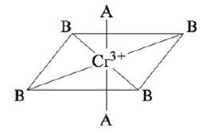Chapter 21, Problem 90CP

Chapter
Section
Textbook Problem

# Consider the pseudo-octahedral complex ion of Cr3+, where A and B represent ligands.Ligand A produces a stronger crystal field than ligand B. Draw an appropriate crystal field diagram for this complex ion (assume the A ligands are on the z-axis).

Interpretation Introduction

Interpretation: An appropriate crystal field diagram for the given complex ion of Cr+3 is to be drawn. Ligand A is considered to be a strong crystal field ligand and it is on the z axis.

Concept introduction: The electrons in the d orbital of a transition metal split into high and low energy orbitals when ligands are attached to it. The energy difference between these two levels depends upon the properties of both metal and the ligands. If the ligand is strong, then splitting will be high and the complex will be low spin. If the ligand is weak, then splitting will be less and the complex will be high spin.

To determine: An appropriate crystal field diagram for the given complex ion of Cr+3 .

Explanation

Explanation

The given complex is,

Figure 1

The central metal is Cr and charge on it is +3 .

The atomic number of Cr is 24 , hence its electronic configuration is [Ar]3d54s1 . With the loss of three electrons, it becomes Cr+3 and its electronic configuration is [Ar]3d3

### Still sussing out bartleby?

Check out a sample textbook solution.

See a sample solution

#### The Solution to Your Study Problems

Bartleby provides explanations to thousands of textbook problems written by our experts, many with advanced degrees!

Get Started